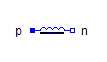Intersective PWM - MapleSim Help

Intersective PWM

Intersective PWMDescription

The Intersective PWM component transforms the input space phasor $u$ to three phase voltages, and compares them with the reference signals. As long as the phase voltage is greater than the corresponding reference signal, the corresponding fire signal is true. The switching pattern of the negative fire signal is the inverse of the positive fire signal. The user can choose from four different reference signals:

 • Sawtooth1: sawtooth signal, same phase in all 3 phases
 • Sawtooth3: sawtooth signal, phase shift between the 3 phases = period/3
 • Triangle1: triangle signal, same phase in all 3 phases
 • Triangle3: triangle signal, phase shift between the 3 phases = period/3Connections

 Name Description Modelica ID $u$ Reference space phasor u ${\mathrm{fire}}_{p}$ positive fire signal fire_p ${\mathrm{fire}}_{n}$ negative fire signal fire_nParameters

 Name Default Units Description Modelica ID $f$ $\mathrm{Hz}$ Switching frequency f ${T}_{0}$ $0$ $s$ Start time of PWM startTime ${u}_{\mathrm{max}}$ Maximum amplitude of signal uMax ref type  Type of reference signal refType

 $\mathrm{Modelica}\cdot \mathrm{Electrical}\cdot \mathrm{PowerConverters}\cdot \mathrm{Types}\cdot \mathrm{ReferenceType}\cdot \mathrm{Triangle3}$Modelica Standard Library The component described in this topic is from the Modelica Standard Library. To view the original documentation, which includes author and copyright information, click here.